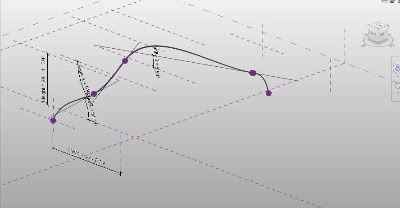## Friday, October 23, 2009

### Tutorial - Revit and Calculus - Part 1My Calculus 1 professor has given us the option of doing a final project instead of taking a final exam. Given the hatred I have for any type of testing I decided to start tackling the idea of a final project. One thing that has always fascinated me about Revit families is the ability to use formulas.

The ability to create parametric families is already a major advantage but using formulas to drive some of these parameters opens up a whole new world. First, I would like to copy and paste the page in "Revit Help" that presents the correct syntax to use when conducting formulas within Revit:

"Formulas support the following arithmetic operations: addition, subtraction, multiplication, division, exponentiation, logarithms, and square roots. Formulas also support the following trigonometric functions: sine, cosine, tangent, arcsine, arccosine, and arctangent.
The valid formula abbreviations for arithmetic operations and trigonometric functions are

• Subtraction— -
• Multiplication—*
• Division—/
• Exponentiation—^: x^y, x raised to the power of y
• Logarithm—log
• Square root—sqrt: sqrt(16)
• Sine—sin
• Cosine—cos
• Tangent—tan
• Arcsine—asin
• Arccosine—acos
• Arctangent—atan
• e raised to an x power—exp
• Absolute Value—abs

You can enter integers, decimals, and fractional values in formulas, using normal mathematical syntax, as shown in the examples below:

• Length = Height + Width + sqrt(Height*Width)
• Length = Wall 1 (11000mm)+ Wall 2 (15000mm)
• Area = Length (500mm) * Width (300mm)
• Volume = Length (500mm) * Width (300mm) * Height (800 mm)
• Width = 100m * cos(angle)
• x = 2*abs(a) + abs(b/2)
• ArrayNum = Length/Spacing.

Parameter names in formulas are case sensitive. For example, if a parameter name begins with a capital letter, such as Width, you must enter it in the formula with an initial capital letter. If you enter it in a formula using lower-case letters instead, for example, width * 2, the software will not recognize the formula."

Now, my goal is to create some sort of element, family, or project that will be driven solely by calculus, trigonometry, and basic mathematics. The purpose is to prove the use of these subjects within my field of study (architecture). Therefore, how would architecture and calculus relate?

Topics Covered:
• Creating a Conceptual Mass.
• Create From with Spline and Profile.
• Edit and Create formulas within Families.
• Drive formulas with parameters.